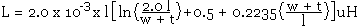# Flat Wire Inductor Calculator

The inductance of a flat or ribbon wire (rectangular cross section), can be found using the calculator or the equation given below.

 INPUT DATA Length: cm Width: cm Thickness: mm
 RESULTS Inductance: nH

This calculator uses JavaScript and will function in most modern browsers.

The inductance of a flat or ribbon wire or a track on a PCB can be calculated with the following equation.Where l is the Length in cm

w is the Width in cm

t is the thickness in cm (PCB copper @ 1 oz/ft2 = 0.035mm)

The original for this equation is by F.E. Terman and can be found in the Radio Engineers Handbook, McGraw-Hill, New York, 1945. The units in the calculator have been changed from µH to nH and the thickness from cm to mm for easier working on PCB's.